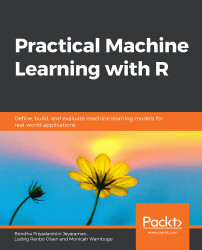•#### Practical Machine Learning with R#### Overview of this book

With huge amounts of data being generated every moment, businesses need applications that apply complex mathematical calculations to data repeatedly and at speed. With machine learning techniques and R, you can easily develop these kinds of applications in an efficient way. Practical Machine Learning with R begins by helping you grasp the basics of machine learning methods, while also highlighting how and why they work. You will understand how to get these algorithms to work in practice, rather than focusing on mathematical derivations. As you progress from one chapter to another, you will gain hands-on experience of building a machine learning solution in R. Next, using R packages such as rpart, random forest, and multiple imputation by chained equations (MICE), you will learn to implement algorithms including neural net classifier, decision trees, and linear and non-linear regression. As you progress through the book, you’ll delve into various machine learning techniques for both supervised and unsupervised learning approaches. In addition to this, you’ll gain insights into partitioning the datasets and mechanisms to evaluate the results from each model and be able to compare them. By the end of this book, you will have gained expertise in solving your business problems, starting by forming a good problem statement, selecting the most appropriate model to solve your problem, and then ensuring that you do not overtrain it.Free Chapter
An Introduction to Machine LearningData Cleaning and Pre-processingFeature EngineeringIntroduction to neuralnet and Evaluation MethodsLinear and Logistic Regression ModelsUnsupervised LearningAppendix## Introduction

In this chapter, we will look at the implementation of unsupervised learning. We will explore different ways of clustering; namely, bottom-up (or agglomerative) and top-down (or divisive). We will also look at the distinction between monothetic and polythetic hierarchical clustering and delve deeper into the implementation of k-means, a popular clustering technique.

Before we go into the details of the chapter, let's take a brief look at an overview of machine learning. Machine learning, in general, can be divided into three distinct groups; namely, reinforcement learning, supervised learning, and unsupervised learning, as shown in Figure 6.1. There is also one more category, semi-supervised learning, which falls between supervised learning and unsupervised learning. Most widely used learning techniques are supervised and unsupervised learning.

###### Figure 6.1: Types of machine learning

Reinforcement learning is a category of machine learning that focuses on training...